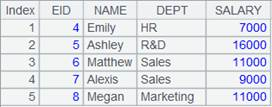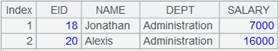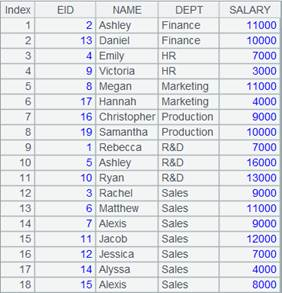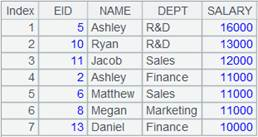# iterate()

## A.iterate()

A.iterate(x,a,c)

 @a 返回序列中每个成员的运算结果

 A 序列/排列 x 表达式 a 初始值 c 返回true/false类型的表达式

x的计算结果

 A 1 [2,222,22,122,2222] 2 =A1.iterate(~*2) 4444 3 =A1.iterate@a(~*2) [4,444,44,244,4444] 4 =A1.iterate(~~*2,3) 96 5 =A1.iterate(~~*2,5,~>500) 80 6 =demo.query("select * from SALES") 7 =A6.derive(A6.(AMOUNT).iterate(~~*2,5,~>20000):Cumulation)iterate()

## ch.iterate()

ch.iterate(x,a,c)

 ch 管道 x 表达式 a 初始值 c 返回true/false类型的表达式

x的计算结果

 A 1 =[2,222,22,122,2222] 2 =channel() 3 =channel() 4 =A2.iterate(~*2) 5 =A3.iterate(~~*2,3) 6 =A1.push(A2,A3) 7 =A2.result() 4444 8 =A3.result() 96 9 =[100,200,800,2000] 10 =channel() 11 =A10.iterate(~~*3,10,~>250) 12 =A9.push(A10) 13 =A10.result() 90

## cs.iterate()

cs.iterate(x,a,c)

 cs 游标 x 表达式 a 初始值 c 返回true/false类型的表达式

x的计算结果

 A 1 =[2,222,22,122,2222].cursor() 2 =A1.iterate(~*2) 4444 3 =[2,222,22,122,2222].cursor().iterate(~~*2,3) 96 4 =[2,222,22,122,2222].cursor().iterate(~~*2,5,~>500) 80 5 =demo.cursor("select * from SALES") 6 =A5.(AMOUNT).iterate(~~*2,5,~>20000) 40

## iterate()

iterate(x,a;Gi,…)

 x 含有~~的表达式 a 初始值 Gi 字段名

x的计算结果

 A 1 =demo.query("select top 7 * from SCORES ") 2 =A1.groups(CLASS:Class,STUDENTID:StudentID;iterate(~~*2): Score1)3 =A1.groups(CLASS:Class,STUDENTID:StudentID;iterate(~~*2,10): Score1)4 =A1.derive(iterate(~~*2,10;STUDENTID):F1)A.iterate()